## Finite Element Basics

45 minutes basic

### Lesson Objectives

Understand a basic finite element discretization of the Poisson equation in MFEM.
Learn how to launch serial and parallel runs of MFEM examples.
Learn how to visualize the results of MFEM simulations.

### Note

Please complete the   Getting Started page before this lesson.

### Poisson equation

The Poisson Equation is a partial differential equation (PDE) that can be used to model steady-state heat conduction, electric potentials, and gravitational fields. In mathematical terms

$$-\Delta u = f$$

where u is the potential field and f is the source function. This PDE is a generalization of the Laplace Equation.

To approximately solve the above continuous equation on computers, we need to discretize it by introducing a finite (discrete) number of unknowns to compute for. In the Finite Element Method (FEM), this is done using the concept of basis functions.

Instead of calculating the exact analytic solution u, we approximate it

$$u \approx u_h := \sum_{j=1}^n c_j \varphi_j$$

where $u_h$ is the finite element approximation with degrees of freedom (unknown coefficients) $c_j$, and $\varphi_j$ are known basis functions. The FEM basis functions are typically piecewise-polynomial functions on a given computational mesh, which are only non-zero on small portions of the mesh.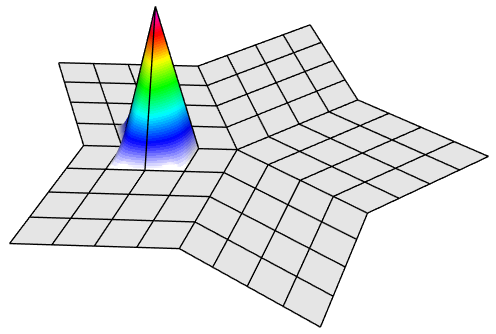With finite elements, the mesh can be totally unstructured, curved, and non-conforming: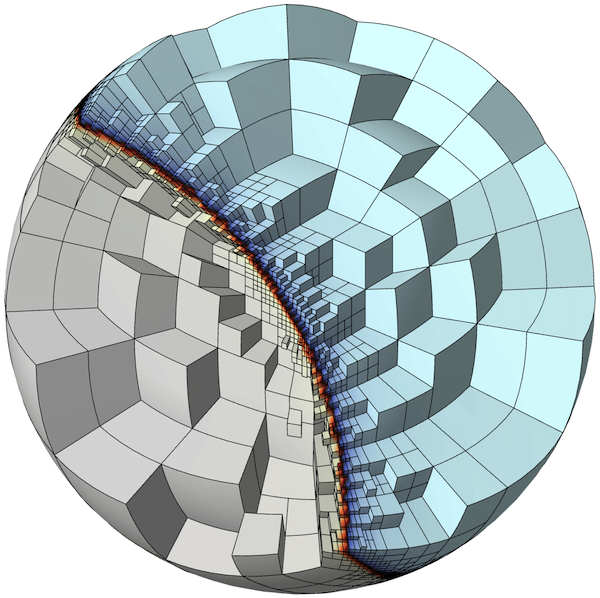To solve for the unknown coefficients, we multiply Poisson's equation by another (test) basis function $\varphi_i$ and integrate by parts to obtain

$$\sum_{j=1}^n\int_\Omega c_j \nabla \varphi_j \cdot \nabla \varphi_i = \int_\Omega f \varphi_i$$

for every basis function $\varphi_i$. (Here we are assuming homogeneous Dirichlet boundary conditions corresponding, for example, to zero temperature on the whole boundary.)

Since the basis functions are known, we can rewrite (3) as

$$A x = b$$

where

$$A_{ij} = \int_\Omega \nabla \varphi_j \cdot \nabla \varphi_i$$

$$b_i = \int_\Omega f \varphi_i$$

$$x_j = c_j$$

This is a $n \times n$ linear system that can be solved directly or iteratively for the unknown coefficients. Note that we are free to choose the computational mesh and the basis functions $\varphi_i$, and therefore the finite space, as we see fit.

### Note

The above is a basic introduction to finite elements in the simplest possible settings. To learn more, you can visit MFEM's Finite Element Method page.

### Annotated Example 1

MFEM's Example 1 implements the above simple FEM for the Poisson problem in the source file examples/ex1.cpp. We set $f=1$ in (1) and enforce homogeneous Dirichlet boundary conditions on the whole boundary.

Below we highlight selected portions of the example code and connect them with the description in the previous section. You can follow along by browsing ex1.cpp in your VS Code browser window. In the settings of this tutorial, the visualization will automatically update in the GLVis browser window.

The computational mesh is provided as input (option -m), it could be 3D, 2D, surface, hex/tet, etc. The following code (lines 120-137) loads the mesh from the given file, mesh_file; creates the corresponding MFEM object mesh of class Mesh; and refines the mesh uniformly to about 50,000 elements. You can easily modify the refinement by changing the definition of ref_levels.

Mesh mesh(mesh_file, 1, 1);
int dim = mesh.Dimension();

int ref_levels = (int)floor(log(50000./mesh.GetNE())/log(2.)/dim);
for (int l = 0; l < ref_levels; l++)
{
mesh.UniformRefinement();
}


In the next section we create the finite element space, i.e., specify the finite element basis functions $\varphi_j$ on the mesh. This involves the MFEM classes FiniteElementCollection, which specifies the space (including its order, provided as input via -o), and FiniteElementSpace, which connects the space and the mesh.

Focusing on the common case order > 0, the code in lines 139-162 is essentially:

FiniteElementCollection *fec = new H1_FECollection(order, dim);
FiniteElementSpace fespace(&mesh, fec);
cout << "Number of finite element unknowns: " << fespace.GetTrueVSize() << endl;


The printed number of finite element unknowns (typically) corresponds to the size of the linear system $n$ from the previous section.

The finite element degrees of freedom that are on the domain boundary are then extracted in lines 164-174. We need those to impose the Dirichlet boundary conditions.

Array<int> ess_tdof_list;
if (mesh.bdr_attributes.Size())
{
Array<int> ess_bdr(mesh.bdr_attributes.Max());
ess_bdr = 1;
fespace.GetEssentialTrueDofs(ess_bdr, ess_tdof_list);
}


The method GetEssentialTrueDofs takes a marker array of Mesh boundary attributes and returns the FiniteElementSpace degrees of freedom that belong to the marked attributes (the non-zero entries of ess_bdr).

The right-hand side $b$ is constructed in lines 176-182. In MFEM terminology, integrals of the form (6) are implemented in the class LinearForm. The Coefficient object corresponds to $f$ from the previous section, which here is set to $1$. You can easily specify more general $f$ with other coefficient classes, e.g., FunctionCoefficient.

LinearForm b(&fespace);
ConstantCoefficient one(1.0);
b.Assemble();


The finite element approximation $u_h$ is described in MFEM as a GridFunction belonging to the FiniteElementSpace. Note that a GridFunction object can be viewed both as the function $u_h$ in (2) as well as the vector of degrees of freedom $x$ in (7). See lines 184-188.

GridFunction x(&fespace);
x = 0.0;


We need to initialize x with the boundary values we want to impose as Dirichlet boundary conditions (for simplicity, here we just set x=0 in the whole domain).

The matrix $A$ is represented as a BilinearForm object, with a specific DiffusionIntegrator corresponding to the weak form (5). See lines 190-203.

BilinearForm a(&fespace);
if (pa) { a.SetAssemblyLevel(AssemblyLevel::PARTIAL); }
if (fa) { a.SetAssemblyLevel(AssemblyLevel::FULL); }
a.Assemble();


MFEM supports different assembly levels for $A$ (from global matrix to matrix-free) and many different integrators. You can also provide a variety of coefficients to the integrator, for example, PWConstCoefficient to specify different material properties in different portions of the domain.

The linear system (4) is formed in lines 205-207 and solved with a variety of options in lines 211-245. One simple case is:

OperatorPtr A;
Vector B, X;

a.FormLinearSystem(ess_tdof_list, x, b, A, X, B);
cout << "Size of linear system: " << A->Height() << endl;

GSSmoother M((SparseMatrix&)(*A));
PCG(*A, M, B, X, 1, 200, 1e-12, 0.0);


The method FormLinearSystem takes the BilinearForm, LinearForm, GridFunction, and boundary conditions (i.e., a, b, x, and ess_tdof_list); applies any necessary transformations such as eliminating boundary conditions (specified by the boundary values of x, applying conforming constraints for non-conforming AMR, static condensation, etc.); and produces the corresponding matrix $A$, right-hand side vector $B$, and unknown vector $X$.

In the above example, we then solve A X = B with a conjugate gradient iteration, using a simple Gauss-Seidel preconditioner. We set the maximum number of iterations to 200 and a convergence criteria of residual norm reduction by 6 orders of magnitude (1e-12 is the square of that relative tolerance).

Solving the linear system is one of the main computational bottlenecks in the FEM. It can take many preconditioned conjugate gradient (PCG) iterations depending on the problem size, the difficulty of the problem, and the choice of the preconditioner.

Once the linear system is solved, we recover the solution as a finite element grid function, and then visualize and save the final results to disk (files refined.mesh and sol.gf). See lines 247-267.

a.RecoverFEMSolution(X, b, x);

ofstream mesh_ofs("refined.mesh");
mesh.Print(mesh_ofs);
ofstream sol_ofs("sol.gf");
x.Save(sol_ofs);

socketstream sol_sock("localhost", 19916);
sol_sock << "solution\n" << mesh << x << flush;


### Parallel Example 1p

Like most MFEM examples, Example 1 has also a parallel version in the source file examples/ex1p.cpp, which illustrates the ease of transition between sequential and MPI-parallel code. The parallel version supports all options of the serial example, and can be executed on varying numbers of MPI ranks, e.g., with mpirun -np. Besides MPI, in parallel we also depend on METIS for mesh partitioning and hypre for solvers.

The differences between the two versions are small, and you can compare them for yourself by opening both files in your VS Code window.

The main additions in ex1p.cpp compared to ex1.cpp are:

Initializing MPI and hypre

Mpi::Init();
Hypre::Init();


Splitting the serial mesh in parallel with additional parallel refinement

ParMesh pmesh(MPI_COMM_WORLD, mesh);
int par_ref_levels = 2;
for (int l = 0; l < par_ref_levels; l++)
{
pmesh.UniformRefinement();
}


Using the Par-prefixed versions of the classes

ParFiniteElementSpace fespace(&pmesh, fec);
ParLinearForm b(&fespace);
ParGridFunction x(&fespace);
ParBilinearForm a(&fespace);


Parallel PCG with hypre's algebraic multigrid BoomerAMG preconditioner

Solver *prec = new HypreBoomerAMG;
CGSolver cg(MPI_COMM_WORLD);
cg.SetRelTol(1e-12);
cg.SetMaxIter(2000);
cg.SetPrintLevel(1);
cg.SetPreconditioner(*prec);
cg.SetOperator(*A);
cg.Mult(B, X);


### Note

Unlike in the serial version, we expect the number of PCG iterations to remain relatively bounded with the BoomerAMG preconditioner independent of the mesh size, coefficient jumps, and number of MPI ranks. Note, however, that algebraic multigrid has a non-trivial setup phase, which can be comparable in terms of time with the PCG solve phase. For more details, see the   Solvers and Scalability page.

### Serial and parallel runs

Both ex1 and ex1p come pre-built in the tutorial environment. You can see a number of sample runs at the beginning of their corresponding source files when you open them in VS Code.

To get a feel for how these examples work, you can copy and paste some of these runs from the source to the terminal in VS Code. For example try these runs in the VS Code terminal.

./ex1 -m ../data/escher.mesh
./ex1 -m ../data/l-shape.mesh
./ex1 -m ../data/mobius-strip.mesh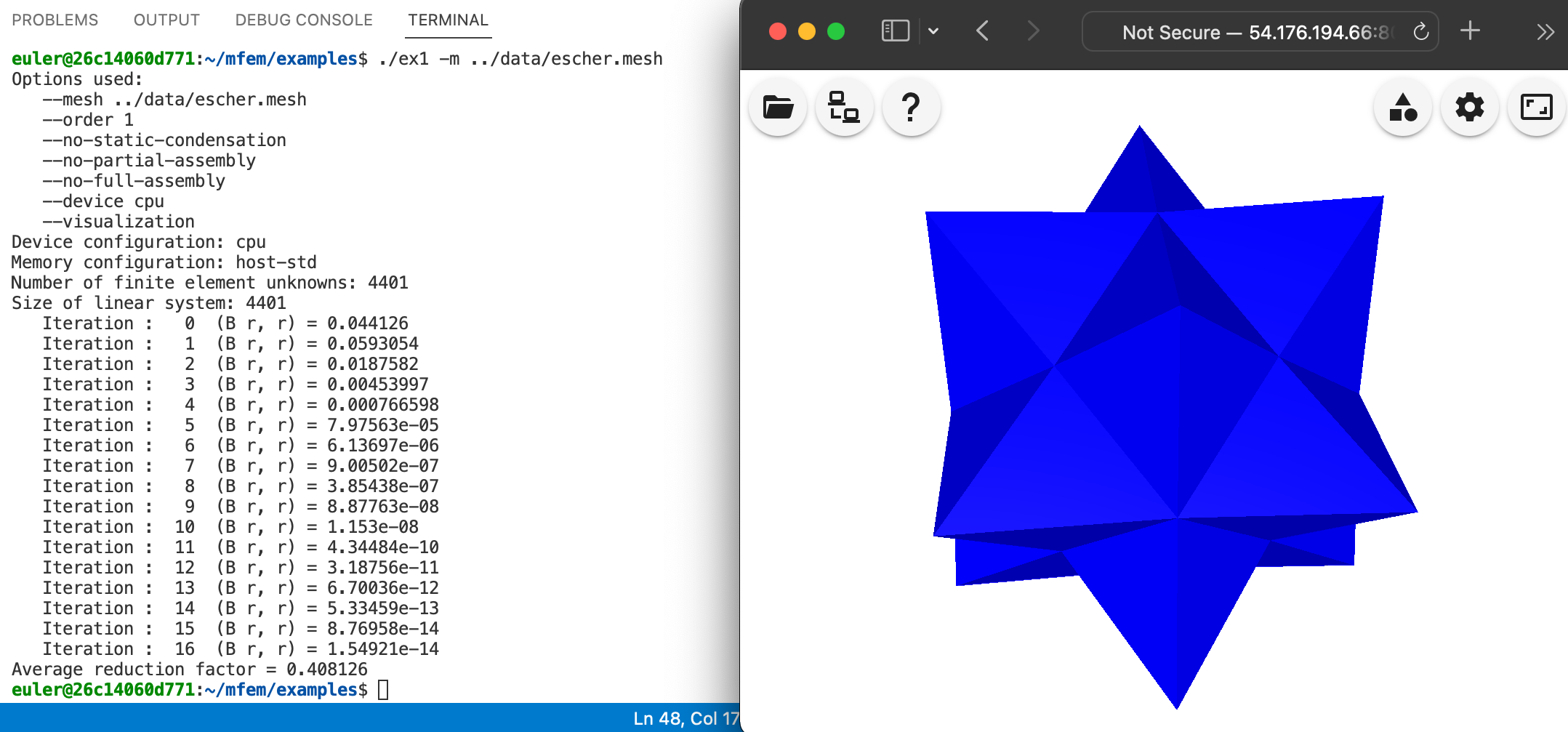### Warning

The current directory is not in the VS Code PATH so make sure to add ./ before the executable, e.g., ./ex1 -m ../data/pipe-nurbs.mesh not ex1 -m ../data/pipe-nurbs.mesh.

### Note

The GLVis visualization is local to your browser, so it may take a while to update after a sample run. Once the data arrives, interaction with the visualization window should be fast.

Here are some sample parallel runs:

mpirun -np 16 ex1p
mpirun -np 16 ex1p -m ../data/pipe-nurbs.mesh
mpirun -np 48 ex1p -m ../data/escher-p2.mesh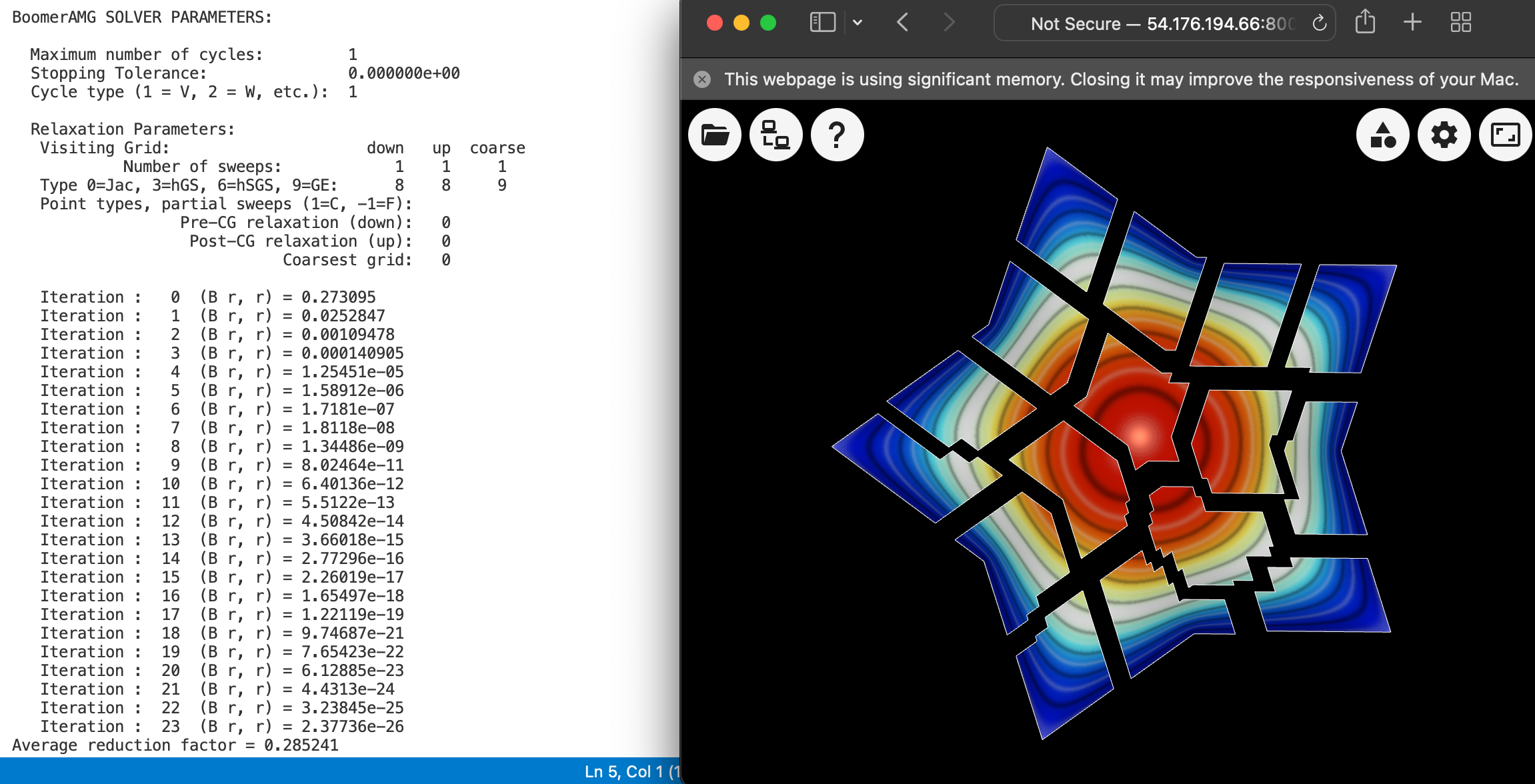The above plot shows the parallel decomposition in the first sample run, with the following manipulations in the GLVis window: pressing keys R, j, b, g, F11 twice, p a number of times, and zooming in with the Right mouse button.

### GLVis interface

GLVis is a lightweight tool for accurate and flexible finite element visualization based on MFEM. In this tutorial we use its web version, which should work on any machine with a modern browser, including mobile touch devices such as tablets and phones.

### Note

The GLVis and VS Code browser windows do not need to be on the same device. For example, you can run VS Code on a computer, while GLVis shows the results on your phone/tablet.

GLVis natively understands finite element data and can manipulate it in various ways through the web interface or by typing (case sensitive) keystrokes in the GLVis window.

To access the web interface, move to the top right of the GLVis window and press the Visualization controls icon . This will open a number of buttons for controlling the mesh, colors, and position of the plot: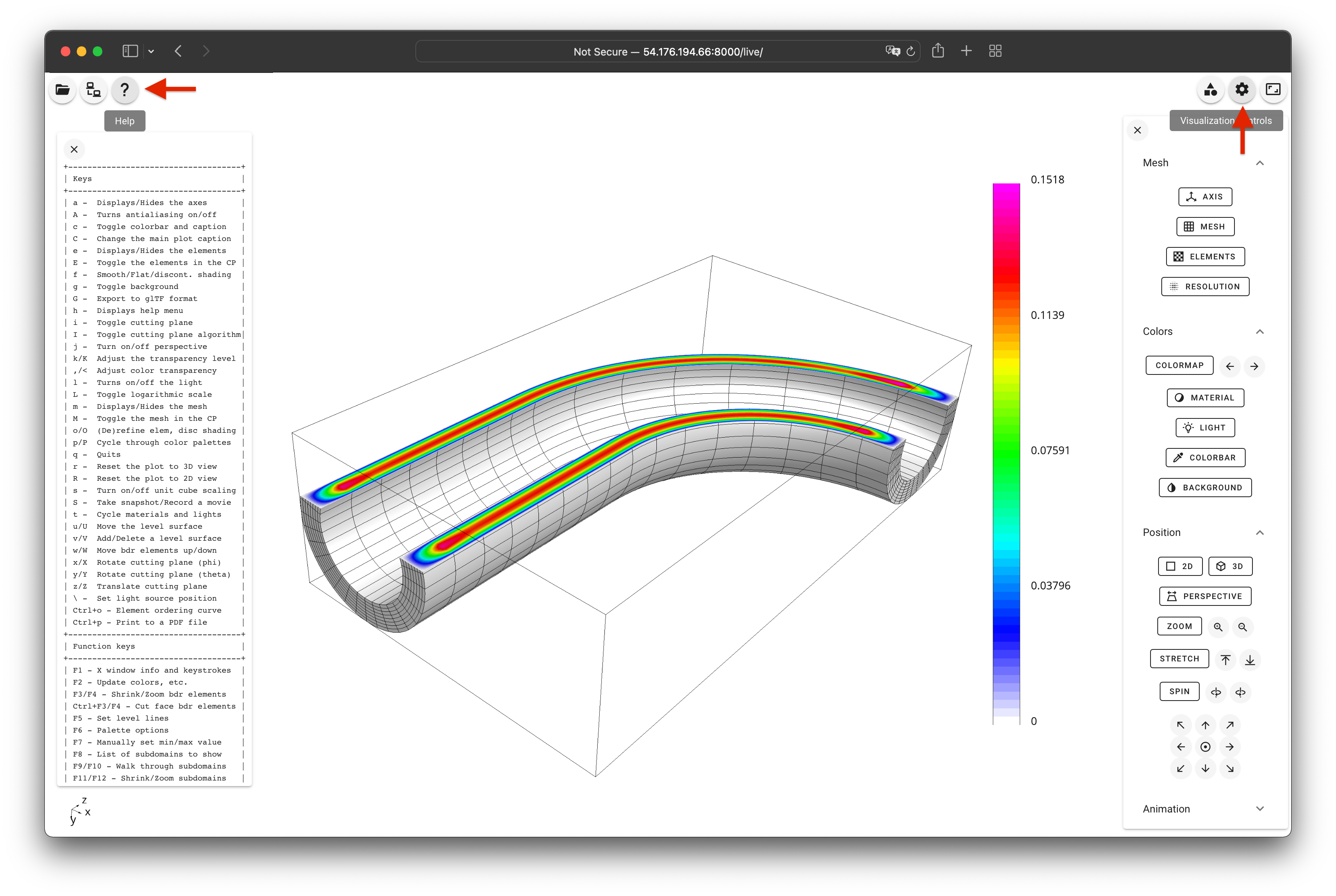You can perform additional operations with the GLVis key commands and mouse functions. Most of them are described in the Help window that appears when clicking theicon in the upper left corner, or by pressing the h key.

Some of the more useful key commands and mouse functions are:

• A — Turn on/off the use of anti-aliasing/multi-sampling
• b — Toggle the boundary in 2D scalar mode
• c — Show/hide color bar
• F11 / F12 — Shrink/Zoom parallel subdomains
• g — Toggle background color (white/black)
• i — Toggle cutting plane
• j — Turn on/off perspective
• Left — Rotate the plot
• Left + Shift — Spin the plot (according to the dragging vector)
• m — Toggle the mesh state.
• p / P — Cycle through color palettes (lots of options)
• r — Reset the plot to 3D view
• R — Cycle through 2D projections (looking above/below in x/y/z directions)
• Right — Zoom in/out
• S — Take an image snapshot
• space — Pause solution update in time-dependent simulations
• t — Cycle materials and lights
• x / X — Rotate cutting plane (\phi) in 3D
• y / Y — Rotate cutting plane (\theta) in 3D
• z / Z — Translate cutting plane in 3D

Note that you may need to press fn and/or Ctrl to escape some of the function keys.

For more details, see the full list of key commands and mouse functions in the GLVis README.

### Warning

If the GLVis window becomes unresponsive, try disconnecting and connecting again. If this doesn't help, run the following in the VS Code terminal: pkill -f glvis-browser-server, then force-reload the GLVis browser window and connect again.

### Questions?

Ask for help in the tutorial Slack channel.

### Next Steps

Depending on your interests pick one of the following lessons:

Back to the MFEM tutorial page